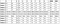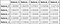# How we deal with sparse data at SentinelOne

At SentinelOne, we work with a lot of sparse data. Our static engine uses many features about files on the client’s computer. We scan those files and extract a numeric array to feed into our models. That data tends to be sparse. Luckily, `numpy` and `scipy` have many great features for working with sparse data, but sometimes, we need a little extra.

# Spartans

We started writing more and more functions and methods, which we eventually packaged together to create Spartans SPARse Tools for ANalysis. Spartans allows you to have a rich feature set of functions to work on and with you sparse matrices. It also has a (beta) FeatureMatrix — a sparse matrix object that has feature names and works with OOB algorithms from `sklearn` and the such.

`import spartansimport pandas as pdimport numpy as npfrom scipy import sparse`

# Our Main Goal in Mind

At some point, we understood that our models performed really well, but we wanted to understand our features a little better. We want a real human understanding of our features and not just their mathematical effect. We started by wanting to create a simple correlation plot. In `pandas` this would be easy to achieve:

`df = pd.read_csv('data/data.csv',index_col=0)`DataFrame with some generated data

cor = df.corr()
cordf.corr() applied on a DataFrame

Alas, sparse matrices give us a harder time. calling `pd.corr` or `np.corrcoef` will result in an error.

# Basic Building Block

`numpy` does give us an efficient method to extract the mean, with `np.mean` , which luckily works with sparse matrices.

`m = np.array([[1, -2, 0, 50, 1],              [0, 0, 0, 100, 0],              [1, 0, 0, 80, 0],              [1, 4, 0, 0, 0],              [0, 0, 0, 0, 0],              [0, 4, 0, 0, 0],              [0, 0, 0, -50, 0]])c = sparse.csr_matrix(m)c`

Out:

`<7x5 sparse matrix of type '<class 'numpy.int64'>'	with 11 stored elements in Compressed Sparse Row format>`

In :

`np.mean(m, axis=0)`

Out:

`array([ 0.4286,  0.8571,  0.    , 25.7143,  0.1429])`

In :

`np.mean(c, axis=0)`

Out:

`matrix([[ 0.4286,  0.8571,  0.    , 25.7143,  0.1429]])`

# University Pays Off

`numpy` cannot compute the variance for sparse matrices out of the box. But we know this little trick:

It may look complex writing as formulas, but it’s actually pretty easy in code.

`X2  = c.power(2)EX  = np.mean(c,axis=0)EX2 = np.mean(X2, axis=0)E2X = np.power(EX,2)V = EX2 - E2X`

We incorporated all of those into Spartans and now we just use `spartans.variance(c, axis=0)` . And yes, like in `numpy` we can compute everything on each of the (two) axes.

# Correlation Matrix

Our objective was to compute the correlation matrix. For this we need to compute the covariance, and the covariance matrix first. `spartans` provides us with those methods.

In :

`spartans.cov(c)`

Out:

`matrix([[   0.2449,   -0.0816,    0.    ,    7.551 ,    0.0816],        [  -0.0816,    4.4082,    0.    ,  -36.3265,   -0.4082],        [   0.    ,    0.    ,    0.    ,    0.    ,    0.    ],        [   7.551 ,  -36.3265,    0.    , 2395.9184,    3.4694],        [   0.0816,   -0.4082,    0.    ,    3.4694,    0.1224]])`

From here, it is easy to get the correlation matrix, by dividing by the variance of each row and column.

`fig, ax = plt.subplots(figsize=(10,10))plt.imshow(np.abs(spartans.corr(c)), vmin=0,vmax=1, cmap='Purples')plt.colorbar()ax.grid()`

Have you noticed the grey row and column? The correlation of feature 2 is `np.nan` with each of the other variables. If you look again, you see that feature is a constant feature with variance of 0.

# Indexing

`spartans` provides has easy methods for indexing. A common problem for sparse matrices is that sometimes entire columns are zero. We’ll get rid of those using `spartans.non_zero_index(c, axis=0, as_bool=False)` which will give us `array([0, 1, 3, 4])` . Then we’ll slice accordingly :

In :

`C = c[:,spartans.non_zero_index(c, axis=0, as_bool=False)].todense()C`

Out:

`matrix([[  1,  -2,  50,   1],        [  0,   0, 100,   0],        [  1,   0,  80,   0],        [  1,   4,   0,   0],        [  0,   0,   0,   0],        [  0,   4,   0,   0],        [  0,   0, -50,   0]], dtype=int64)`

We may see some columns that are non-zero but constant. Or maybe they are almost constant with only a few non zero values. For example, the last column has only 1 non zero.

In :

`spartans.non_constant_index(c,axis=0, threshold=1,method='nnz') # Faster`

Out:

`array([ True,  True, False,  True, False])`

In :

`spartans.non_constant_index(c,axis=0,threshold=1/7,method='variance')`

Out:

`array([ True,  True, False,  True, False])`

# Masking

One of the biggest problems working with sparse matrices is null values. Many algorithms tend to mix between the native zeros of the matrix and the missing values. In `spartans` we have masking. Masking creates an accompanying boolean sparse matrix pointing at the missing values. Then we can use those in later computations or by stacking it together with the original matrix if we believe this information is valuable.

In :

`c_with_mask.todense()`

Out:

`matrix([[  1.,  -2.,   0.,  50.,   1.],        [  0.,   0.,   0., 100.,   0.],        [  1.,   0.,   0.,  80.,  nan],        [  1.,   4.,   0.,   0.,   0.],        [  0.,   0.,   0.,   0.,   0.],        [  0.,   4.,   0.,   0.,   0.],        [  0.,   0.,   0., -50.,   0.]], dtype=float32)`

In :

`msk = spartans.make_nan_mask(c_with_mask)print (repr(msk))print (msk)print (msk.todense())>>><7x5 sparse matrix of type '<class 'numpy.bool_'>'	with 1 stored elements in Compressed Sparse Column format>  (2, 4)	True[[False False False False False] [False False False False False] [False False False False  True] [False False False False False] [False False False False False] [False False False False False] [False False False False False]]`

`spartans `is a great open-source package. It bridged the gap between operation you are used to with dense arrays and sparse matrices. Its main functionalities are everyday mathematical tasks like computing the variance, finding covariances creating a correlation matrix. Moreover, `spartans` lets you index your matrix by several conditions that may be of interest when preparing a dataset for machine learning tasks. It also enables you to differentiate between “real zeros” in your data and between missing data.

Try Spartans- https://github.com/Sentinel-One/Spartans

This post was written by Dean Langsam, Data Scientist at SentinelOne.

You can follow Dean on his personal blog www.deanla.com , or even better- join him! We’re hiring.

This is the tech blog of Sentinelone, a leading cybersecurity company. Follow us to learn about the awesome tech we build here.

This is the tech blog of Sentinelone, a leading cybersecurity company. Follow us to learn about the awesome tech we build here.